# MP Board Class 6th Maths Solutions Chapter 7 Fractions Ex 7.4

## MP Board Class 6th Maths Solutions Chapter 7 Fractions Ex 7.4

Question 1.
Write shaded portion as fraction. Arrange them in ascending and descending order using correct sign ‘<‘ ‘=’ ‘>’ between the fractions: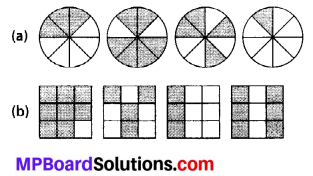(c) Show $$\frac{2}{6}, \frac{4}{6}, \frac{8}{6}$$ and $$\frac{6}{6}$$ on the number line. Put appropriate signs between the fractions given.Solution: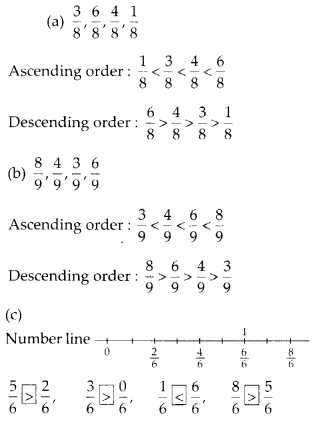Question 2.
Compare the fractions and put an appropriate sign.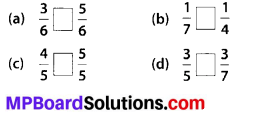Solution:
(a)
$$\frac{3}{6}$$ and $$\frac{5}{6}$$ are like fractions.
Also, denominator of $$\frac{5}{6}$$ is greater than denominator of $$\frac{3}{6}$$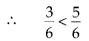(b)
$$\frac{1}{7}$$ and $$\frac{1}{4}$$ are unlike fractions with same numerator. Also, denominator of $$\frac{1}{7}$$ is greater than denominator of $$\frac{1}{4}$$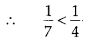(c)
$$\frac{4}{5}$$ and $$\frac{5}{5}$$ are like fractions.
Also, numerator of $$\frac{5}{5}$$ is greater than numerator of $$\frac{4}{5}$$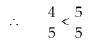(d) $$\frac{3}{5}$$ and $$\frac{3}{7}$$ are unlike fractions with same numerator. Also, denominator of $$\frac{3}{7}$$ is greater than denominator of $$\frac{3}{5}$$.Question 3.
Make five more such pairs and put appropriate signs.
Solution: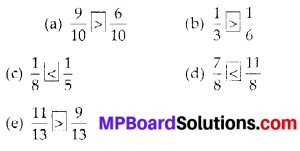Question 4.
Look at the figures and write ‘<‘ or ‘>’, ‘=’ between the given pairs of fractions.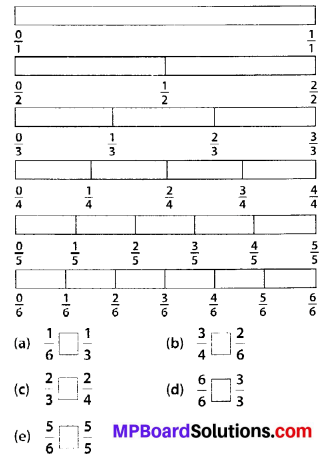make five more such problems and solve them with your friends.
Solution: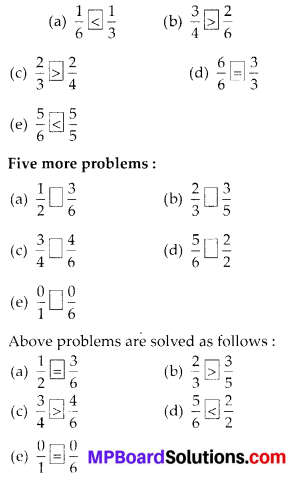Question 5.
How quickly can you do this ? Fill appropriate sign (‘<‘, ‘=’, ‘>’)Solution:
(a) $$\frac{1}{2}$$ and $$\frac{1}{5}$$ are unlike fractions with same numerator. Also denominator of $$\frac{1}{5}$$ is greater than denominator of $$\frac{1}{2}$$.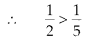(b) $$\frac{2}{3}$$ and $$\frac{3}{6}$$ are unlike fractions with different numerator.(c) $$\frac{3}{5}$$ and $$\frac{2}{3}$$ are unlike fractions with different numerator.(d) $$\frac{3}{4}$$ and $$\frac{2}{8}$$ are unlike fractions with different numerators.(e) $$\frac{3}{5}$$ and $$\frac{6}{5}$$ are like fractions. Also, numerator of $$\frac{6}{5}$$ is greater than numerator of $$\frac{3}{9}$$.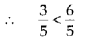(f) $$\frac{7}{9}$$ and $$\frac{3}{9}$$ are like fractions. Also, numerator of $$\frac{7}{9}$$ is greater than numerator of $$\frac{3}{9}$$.(g) $$\frac{1}{4}$$ and $$\frac{1}{2}$$ are unlike fractions with different numerators.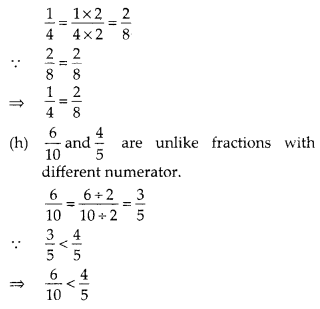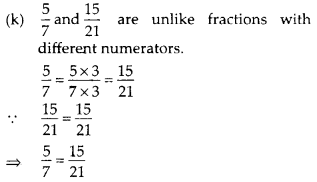Question 6.
The following fractions represent just three different numbers. Separate them into three groups of equivalent fractions, by changing each one to its simplest form.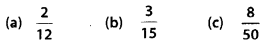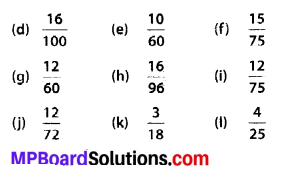Solution:Question 7.
Find answers to the following. Write and indicate how you solved them.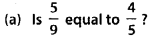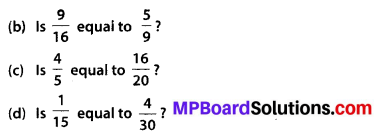Solution: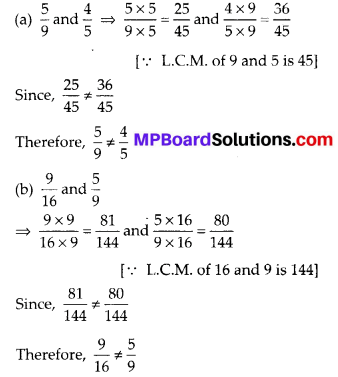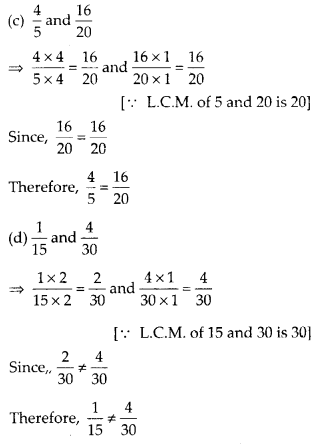Question 8.
Ila read 25 pages of a book containing 100 pages. Lalita read $$\frac{2}{5}$$ of the same book. Who read less ?
Solution:
Ila read 25 pages out of 100 pages.Question 9.
Rafiq $$\frac{3}{6}$$ of an hour, while Rohit exercised for $$\frac{3}{4}$$ of an hour. Who exercised for a longer time?
Solution:
Rafiq exercised $$\frac{3}{6}$$ of an hour.
Rafiq exercised $$\frac{3}{4}$$ of an hour.
Since, $$\frac{3}{4}>\frac{3}{6}$$
Therefore, Rohit exercised for a longer time.Question 10.
In a Class A of 25 students, 20 passed in first class; in another class B of 30 students, 24 passed in first class. In which class was a greater fraction of students getting first class?
Solution:
In class A, 20 passed in first class out of 25.
∴ Fraction of first class passed students $$=\frac{20}{25}=\frac{4}{5}$$
In class B, 24 passed in first class out of 30.
∴ Fraction of first class passed students $$=\frac{24}{30}=\frac{4}{5}$$
Hence, both classes have same fraction of student getting first class.